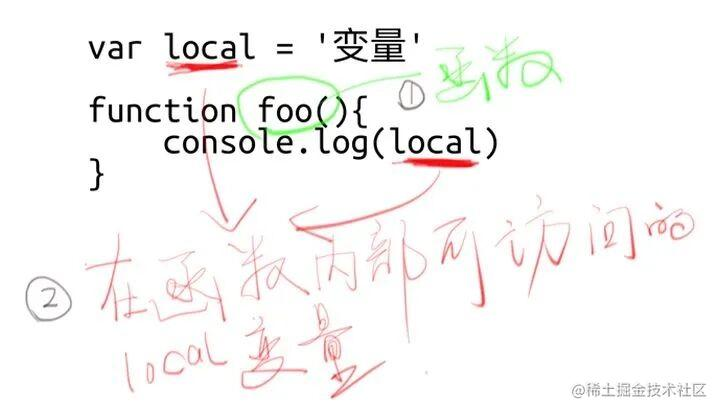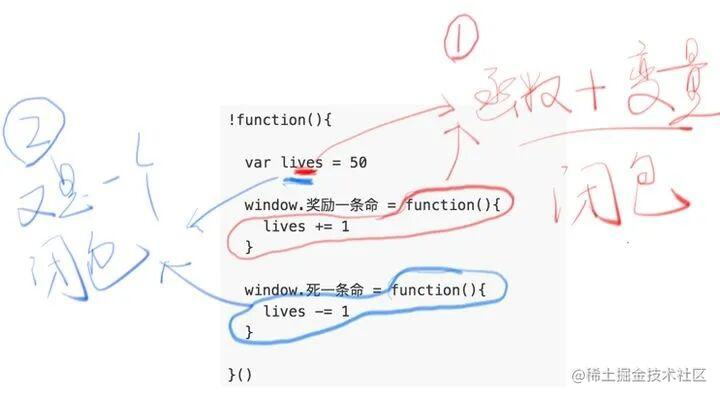ZJ.cao

3.3万nut96yxcKirin_GTTheShyFanchunlurenBin.lzs0511cyb-包子

ZJ.cao
13天前
/**
* @param {string} s
* @param {string} goal
* @return {boolean}
*/
var buddyStrings = function(s, goal) {
const n = s.length,m = goal.length;
let cnt=0,s1,s2,g1,g2;
const map = new Map();
if(n != m) return false;
if(s == goal){
for(let i = 0; i < n; i ++) {
map.set(s[i],map.has(s[i])?map.get(s[i])+1:1);
if(map.get(s[i])>=2) return true;
}
return false;
}
for(let i = 0; i < n; i ++) {
if(s[i]!=goal[i]) {
cnt++;
if(cnt == 1){
s1 = s[i];g1=goal[i];
}else if(cnt == 2) {
s2 = s[i];g2=goal[i];
}
}
if(cnt == 3) return false;
}
if(s1==g2&&s2==g1) return true;
return false;
};


ZJ.cao
15天前
/**
* // Definition for a Node.
* function Node(val,children) {
*    this.val = val;
*    this.children = children;
* };
*/

/**
* @param {Node|null} root
* @return {number}
*/
var maxDepth = function(root) {
if(!root) return 0;
let ans = 0;
const queue = [];
queue.push(root);
while(queue.length) {
let size = queue.length;
while (size > 0) {
const node = queue.shift();
const children = node.children;
for (const child of children) {
queue.push(child);
}
size--;
}
ans++;
}
return ans;
};


ZJ.cao
15天前
/**
* @param {number[]} nums
* @return {number}
*/
var findLHS = function(nums) {
const map = new Map();
let ans = 0;
nums.forEach(x=>{
map.set(x,map.has(x)?map.get(x)+1:1);
});
for(let item of map) {
if(map.has(item-1)) {
ans = Math.max(ans,item+map.get(item-1));
}
}
return ans;
};


ZJ.cao
18天前
/**
* @param {number[]} nums
* @return {number[][]}
*/
var threeSum = function(nums) {
const ans = [];
nums.sort((a,b)=>a-b);
for(let i = 0; i < nums.length; i ++) {
//去重
if(i&&nums[i]==nums[i-1]) continue;
for(let j = i+1,k = nums.length-1; j < k; j ++) {
//去重
if(j > i+1&&nums[j]==nums[j-1]) continue;
while(j<k-1&&nums[i]+nums[j]+nums[k-1]>=0) k--;
if(nums[i]+nums[j]+nums[k]==0) {
ans.push([nums[i],nums[j],nums[k]]);
}
}
}
return ans;
};


ZJ.cao
18天前
/**
* @param {string} s
* @param {number} numRows
* @return {string}
*/
var convert = function(s, numRows) {
if(numRows == 1) return s;
let t = 0,flag = true;
let arr = new Array();
for(let i = 0; i < numRows; i ++) arr[i] = new Array();
for(let i = 0; i < s.length; i ++) {
if(flag) {
t ++;
if(t == numRows) flag = false;
}else {
t --;
if(t == 1) flag = true;
}
arr[t-1].push(s[i]);
}
let ans="";
for(let i = 0; i < numRows; i ++) {
for(let j = 0; j < arr[i].length; j ++) {
ans+=arr[i][j];
}
}
return ans;
};


ZJ.cao
19天前
/**
* @param {string[]} words
* @return {number}
*/
var maxProduct = function(words) {
const len = words.length;
let bits = new Array(len).fill(0);
for(let i = 0; i < len; i++){
for(let char of words[i]){
bits[i] |= 1 << (char.charCodeAt() - 97);
}
}

let res = 0;
for(let i = 0; i < len - 1; i++){
for(let j = i+1; j < len; j++) {
if((bits[i] & bits[j]) === 0) {
res = Math.max(res, words[i].length * words[j].length);
}
}
}
return res;
};


ZJ.cao
22天前

1.什么是「闭包」。

2.「闭包」的作用是什么。「函数」和「函数内部能访问到的变量」的总和，就是一个闭包。

「我听说闭包是需要函数套函数，然后 return 一个函数的呀！」

function foo() {
var local = 1;
function bar() {
local++;
return local;
}
return bar;
}
var func = foo();
func();


window.lives = 30 //还剩30条命


!function() {
var lives = 30;

window.奖励一条命 = function() {
lives += 1;
}

window.死一条命 = function() {
lives -= 1;
}
}()ZJ.cao
1个月前

### 一、原型 prototype和__proto__

• 每个对象都有一个__proto__属性指向它的prototype原型对象
• 每个构造函数都有一个prototype原型对象
• prototype原型对象里的constructor指向构造器本身

function Person(name,age) {
this.name = name;
this.age = age;
this.say = function() {
console.log("我会说话");
}
}
var xm = new Star("小明",18);
var xl = new Star("小亮",19);
console.log(xm.say === xl.say); //false


function Person(name,age) {
this.name = name;
this.age = age;
}
Person.prototype.say = function() {
console.log("我会说话");
}
var xm = new Star("小明",18);
var xl = new Star("小亮",19);
console.log(xm.say); //我会说话


console.log(xm.__proto__ === Person.prototype)//true


### 二、原型链JS原型链查找机制

ZJ.cao
2个月前
class Solution {
public int getSum(int a, int b) {
if(a == 0) return b;
if(b == 0) return a;
int sum = a ^ b;
int carry = (a & b) << 1;
return getSum(sum, carry);
}
}


ZJ.cao
2个月前

### 双指针

class Solution {
public String countAndSay(int n) {
StringBuilder ans = new StringBuilder("1");
for(int i = 1; i <= n-1; i ++){
StringBuilder t = new StringBuilder("");
for(int j = 0; j < ans.length(); j ++){
int k = j;
while(k < ans.length() && ans.charAt(k) == ans.charAt(j))  ++k;
t.append(k-j).append(ans.charAt(j));
j = k-1;
}
ans = t;
}
return ans.toString();
}
}